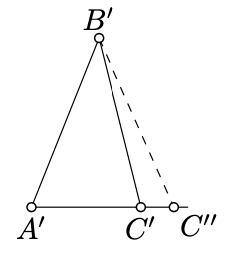$$\newcommand{\id}{\mathrm{id}}$$ $$\newcommand{\Span}{\mathrm{span}}$$ $$\newcommand{\kernel}{\mathrm{null}\,}$$ $$\newcommand{\range}{\mathrm{range}\,}$$ $$\newcommand{\RealPart}{\mathrm{Re}}$$ $$\newcommand{\ImaginaryPart}{\mathrm{Im}}$$ $$\newcommand{\Argument}{\mathrm{Arg}}$$ $$\newcommand{\norm}{\| #1 \|}$$ $$\newcommand{\inner}{\langle #1, #2 \rangle}$$ $$\newcommand{\Span}{\mathrm{span}}$$

# 4.2: Angle-Side-Angle Condition

$$\newcommand{\vecs}{\overset { \rightharpoonup} {\mathbf{#1}} }$$ $$\newcommand{\vecd}{\overset{-\!-\!\rightharpoonup}{\vphantom{a}\smash {#1}}}$$$$\newcommand{\id}{\mathrm{id}}$$ $$\newcommand{\Span}{\mathrm{span}}$$ $$\newcommand{\kernel}{\mathrm{null}\,}$$ $$\newcommand{\range}{\mathrm{range}\,}$$ $$\newcommand{\RealPart}{\mathrm{Re}}$$ $$\newcommand{\ImaginaryPart}{\mathrm{Im}}$$ $$\newcommand{\Argument}{\mathrm{Arg}}$$ $$\newcommand{\norm}{\| #1 \|}$$ $$\newcommand{\inner}{\langle #1, #2 \rangle}$$ $$\newcommand{\Span}{\mathrm{span}}$$ $$\newcommand{\id}{\mathrm{id}}$$ $$\newcommand{\Span}{\mathrm{span}}$$ $$\newcommand{\kernel}{\mathrm{null}\,}$$ $$\newcommand{\range}{\mathrm{range}\,}$$ $$\newcommand{\RealPart}{\mathrm{Re}}$$ $$\newcommand{\ImaginaryPart}{\mathrm{Im}}$$ $$\newcommand{\Argument}{\mathrm{Arg}}$$ $$\newcommand{\norm}{\| #1 \|}$$ $$\newcommand{\inner}{\langle #1, #2 \rangle}$$ $$\newcommand{\Span}{\mathrm{span}}$$

## Theorem $$\PageIndex{1}$$ ASA condition

Assume that

$$AB = A'B'$$, $$\measuredangle ABC = \pm \measuredangle A'B'C'$$, $$\measuredangle CAB = \pm \measuredangle C'A'B'$$

and $$\triangle A'B'C'$$ is nondegenerate. Then

$$\triangle ABC \cong \triangle A'B'C'$$.

Note that for degenerate triangles the statement does not hold. For example, consider one triangle with sides 1, 4, 5 and the other with sides 2, 3, 5.

Proof

According to Theorem 3.3.1, either

$\begin{array} {l} {\meausredangle ABC = \measuredangle A'B'C',} \\ {\measuredangle CAB = \measuredangle C'A'B'} \end{array}$

or

$\begin{array} {l} {\meausredangle ABC = -\measuredangle A'B'C',} \\ {\measuredangle CAB = -\measuredangle C'A'B'.} \end{array}$

Further we assume that 4.2.1 holds; the case 4.2.2 is analogous.Let $$C''$$ be the point on the half-line $$[A'C')$$ such that $$A'C'' = AC$$.

By Axiom IV, $$\triangle A'B'C'' \cong \triangle ABC$$. Applying Axiom IV again, we get that

$$\measuredangle A'B'C'' = \measuredangle ABC = \measuredangle A'B'C'$$.

By Axiom IIIa, $$[B'C') = [BC'')$$. Hence $$C''$$ lies on $$(B'C')$$ as well as on $$(A'C')$$.

Since $$\triangle A'B'C'$$ is not degenerate, $$(A'C')$$ is distinct from $$(B'C')$$. Applying Axiom II, we get that $$C'' = C'$$.

Therefore, $$\triangle A'B'C' = \triangle A'B'C'' \cong \triangle ABC$$.